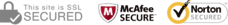The information provided on this section helps to visualise below data based on donut chart.
• Even and Odd set - Any number that can be divided exactly by 2 is an even number. Any number that cannot be divided exactly by 2 is an odd number.
• Low and High set - Any number that ranges from 1 – 18 is a low number. High - Any number that ranges from 19 – 35 is a high number.
• Sequence set - A Sequence is a list of numbers that are in order. Here, we are considering only 2 numbers in sequence.
• Pair set - It is a set of any two numbers.
The colour is based on the random selection.
It shows information on the last 45 draws.
Even Odd Pair: